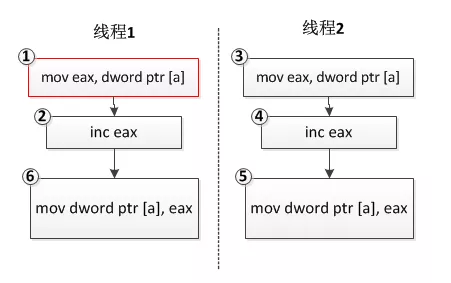• 2021-01-11
• 浏览 (338)

# 整型变量赋值是原子操作吗？

## 整型变量赋值操作不是原子操作

``````int a = 1;
``````

``````mov dword ptr [a], 2
``````

``````a ++;
``````

``````mov eax, dword ptr [a]
inc eax
mov dword ptr [a], eax
``````

``````int a = 0;

//线程1
{
a ++;
}

//线程2
{
a ++;
}
``````• 把一个变量的值赋值给另外一个变量，或者把一个表达式的值赋值给另外一个变量，如
``````  int a = b;
``````

``````  mov eax, dword ptr [b]
mov dword ptr [a], eax
``````